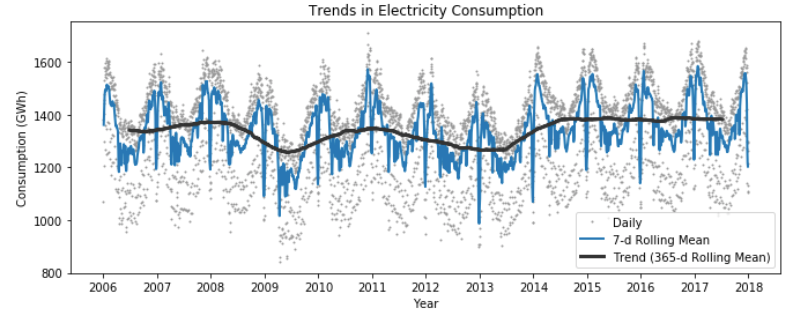1591887180

# Time Series Analysis using Pandas in Python

Right off the bat, time-series data is not your average dataset! You might have worked with housing data wherein each row represents features of a particular house (such as total area, number of bedrooms, year in which it was built) or student dataset wherein each row represents such information about a student (such as age, gender, prior GPA). In all these datasets, the common thing is that all samples (or rows in your dataset), in general, are independent of each other. What sets these datasets apart from time-series data is that in the latter, each row represents a point in time so naturally, there is some inherent ordering to the data. This is how a typical time-series data looks like:

#data-science #python #machine-learning #trend-analysis #time-series-analysis

## Buddha Community1623226129

## Time Series Analysis Using ARIMA Model With Python

Time series_ is a sequence of time-based data points collected at specific intervals of a given phenomenon that undergoes changes over time. In other words, time series is a sequence taken at consecutive equally spaced points in the time period._

As a example, we can present few time series data sets in different domains such as pollution levels, Birth rates, heart rate monitoring, global temperatures and Consumer Price Index etc. At the processing level, above datasets are tracked, monitored, down sampled, and aggregated over time.

There are different kind of time series analysis techniques in the big data analytical field. Among them few are,

• Autoregression (AR)
• Moving Average (MA)
• Autoregressive Moving Average (ARMA)
• Autoregressive Integrated Moving Average (ARIMA)
• Seasonal Autoregressive Integrated Moving-Average (SARIMA)

ARIMA Model

ARIMA Model is simple and flexible enough to capture relationship we would see in the data and It aims to explain the autocorrelation between the data points using past data. We can decompose the ARIMA model as follow to grab the key elements of it.

• **AR: _Auto regression. _**This is a model that uses the dependent relationship between the data and the lagged data.
• **I:_ Integrated. _**The use of differencing of raw observations (e.g. subtracting an observation from an observation at the previous time step) in order to make the time series stationary.
• **MA: _Moving average. _**A model that uses the relationship between the observations and the residual error from the moving average model applied to lagged observations.

Dataset Explanation

Exploratory Analysis

#python #time-series-analysis #pandas #forecasting #arima #time series analysis using arima model with python1619518440

## top 30 Python Tips and Tricks for Beginners

Welcome to my Blog , In this article, you are going to learn the top 10 python tips and tricks.

### 8) Check The Memory Usage Of An Object.

#python #python hacks tricks #python learning tips #python programming tricks #python tips #python tips and tricks #python tips and tricks advanced #python tips and tricks for beginners #python tips tricks and techniques #python tutorial #tips and tricks in python #tips to learn python #top 30 python tips and tricks for beginners1623292080

## Getting started with Time Series using Pandas

### An introductory guide on getting started with the Time Series Analysis in Python

Time series analysis is the backbone for many companies since most businesses work by analyzing their past data to predict their future decisions. Analyzing such data can be tricky but Python, as a programming language, can help to deal with such data. Python has both inbuilt tools and external libraries, making the whole analysis process both seamless and easy. Python’s Panda s library is frequently used to import, manage, and analyze datasets in various formats. However, in this article, we’ll use it to analyze stock prices and perform some basic time-series operations.

#data-analysis #time-series-analysis #exploratory-data-analysis #stock-market-analysis #financial-analysis #getting started with time series using pandas1619510796

## Lambda, Map, Filter functions in python

Welcome to my Blog, In this article, we will learn python lambda function, Map function, and filter function.

Lambda function in python: Lambda is a one line anonymous function and lambda takes any number of arguments but can only have one expression and python lambda syntax is

Syntax: x = lambda arguments : expression

Now i will show you some python lambda function examples:

#python #anonymous function python #filter function in python #lambda #lambda python 3 #map python #python filter #python filter lambda #python lambda #python lambda examples #python map

1586702221

## Python Pandas Objects - Pandas Series and Pandas Dataframe

In this post, we will learn about pandas’ data structures/objects. Pandas provide two type of data structures:-

### Pandas Series

Pandas Series is a one dimensional indexed data, which can hold datatypes like integer, string, boolean, float, python object etc. A Pandas Series can hold only one data type at a time. The axis label of the data is called the index of the series. The labels need not to be unique but must be a hashable type. The index of the series can be integer, string and even time-series data. In general, Pandas Series is nothing but a column of an excel sheet with row index being the index of the series.

### Pandas Dataframe

Pandas dataframe is a primary data structure of pandas. Pandas dataframe is a two-dimensional size mutable array with both flexible row indices and flexible column names. In general, it is just like an excel sheet or SQL table. It can also be seen as a python’s dict-like container for series objects.

#python #python-pandas #pandas-dataframe #pandas-series #pandas-tutorial